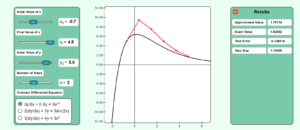# Chapter 08 Simulation of Euler’s Method

Description:  This simulation shows how Euler’s method works to solve ordinary differential equations of the form dy/dx=f(x,y), y(x0)=y0.Keywords: Ordinary differential equation, numerical methods, Euler’s method.

Learning Objectives:  After successful completion of running the simulation with proper questions asked by the instructor, the student would be able to 1) note how the Euler’s method of solving ordinary differential equations works 2) study the effect of step size on the convergence of the method.

Full Resources:  The full resources for the topic include textbook content,  PowerPoint presentations, multiple-choice tests, audiovisual lectures, and application examples.